# Neural networks generalize because of this one weird trick

63 min read18th Jan 20231 comment

# 55

Produced under the mentorship of Evan Hubinger as part of the SERI ML Alignment Theory Scholars Program - Winter 2022 Cohort

A big thank you to all of the people who gave me feedback on this post: Edmund Lao, Dan Murfet, Alexander Gietelink Oldenziel, Lucius Bushnaq, Rob Krzyzanowski, Alexandre Variengen, Jiri Hoogland, and Russell Goyder.

Statistical learning theory is lying to you: "overparametrized" models actually aren't overparametrized, and generalization is not just a question of broad basins.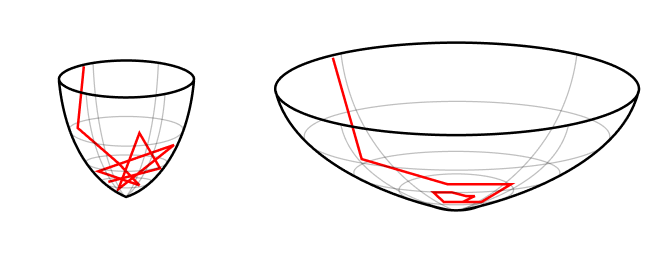The standard explanation thrown around here for why neural networks generalize well is that gradient descent settles in flat basins of the loss function. On the left, in a sharp minimum, the updates bounce the model around. Performance varies considerably with new examples. On the right, in a flat minimum, the updates settle to zero. Performance is stabler under perturbations.

To first order, that's because loss basins actually aren't basins but valleys, and at the base of these valleys lie "rivers" of constant, minimum loss. The higher the dimension of these minimum sets, the lower the effective dimensionality of your model. Generalization is a balance between expressivity (more effective parameters) and simplicity (fewer effective parameters).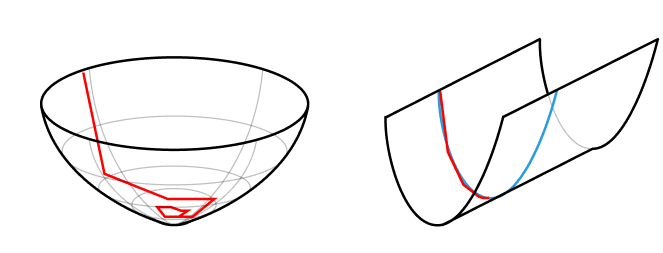Symmetries lower the effective dimensionality of your model. In this example, a line of degenerate points effectively restricts the two-dimensional loss surface to one dimension.

In particular, it is the singularities of these minimum-loss sets — points at which the tangent is ill-defined — that determine generalization performance. The remarkable claim of singular learning theory (the subject of this post), is that "knowledge … to be discovered corresponds to singularities in general" . Complex singularities make for simpler functions that generalize further.The central claim of singular learning theory is that the singularities of the set of minima of the loss function determine learning behavior and generalization. Models close to more complex singularities generalize further.

Mechanistically, these minimum-loss sets result from the internal symmetries of NNs: continuous variations of a given network's weights that implement the same calculation. Many of these symmetries are "generic" in that they are predetermined by the architecture and are always present. The more interesting symmetries are non-generic symmetries, which the model can form or break during training.

In terms of these non-generic symmetries, part of the power of NNs is that they can vary their effective dimensionality. Generality comes from a kind of internal model selection in which the model finds more complex singularities that use fewer effective parameters that favor simpler functions that generalize further.

At the risk of being elegance-sniped, SLT seems like a promising route to develop a better understanding of generalization and the limiting dynamics of training. If we're lucky, SLT may even enable us to construct a grand unified theory of scaling.

A lot still needs to be done (in terms of actual calculations, the theorists are still chewing on one-layer tanh models), but, from an initial survey, singular learning theory feels meatier than other explanations of generalization. It's more than just meatiness; there's a sense in which singular learning theory is a non-negotiable prerequisite for any theory of deep learning. Let's dig in.

# Back to the Bayes-ics

Singular learning theory begins with four things:

• The "truth", , which is some distribution that is generating our samples;
• A model, parametrized by weights , where  is compact;
• A prior over weights, ;
• And a dataset of samples , where each random variable  is i.i.d. according to .

Here, I'm follow the original formulation and notation of Watanabe . Note that most of this presentation transfers straightforwardly from the context of density estimation (modeling ) to other problems like regression and classification (modeling ) . (I also am deeply indebted to Carroll's Msc. Thesis  and the wonderful seminars and notes at metauni )

The lower-level aim of "learning" is to find the optimal weights for the given dataset. As good Bayesians, this has a very specific and constrained meaning:

The higher-level aim of "learning" is to find the optimal model class/architecture, for the given dataset. Rather than try to find the weights that maximize the likelihood or even the posterior, the true aim of a Bayesian is to find the model that maximizes the model evidence,

The fact that the Bayesian paradigm can integrate out its weights to make statements over entire model classes is one of its main strengths. The fact that this integral is often almost always intractable is one of its main weaknesses. So the Bayesians make a concession to the frequentists with a much more tractable Laplace approximation: we find a choice of weights, , that maximizes the likelihood and then approximate the distribution as Gaussian in the vicinity of that point.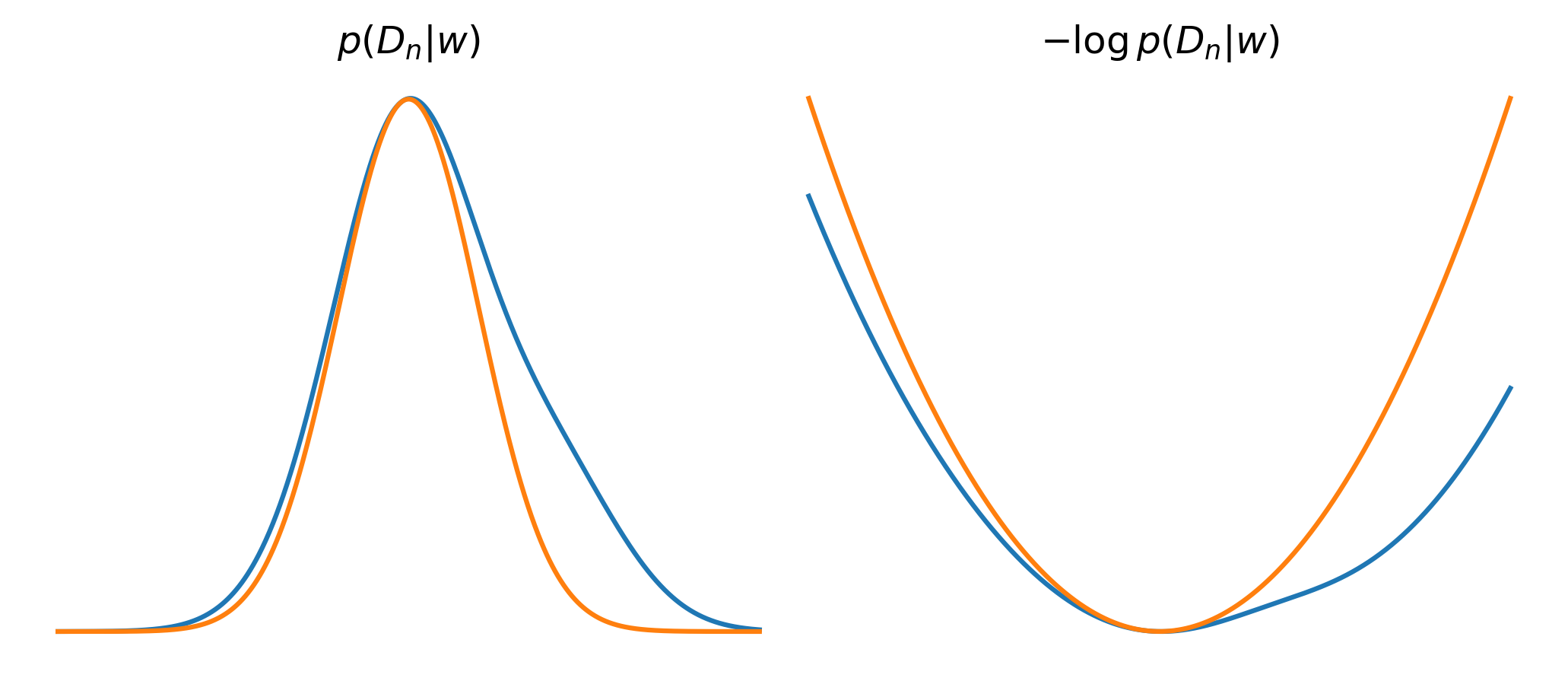The Laplace Approximation is just a probability theorist's (second-order) Taylor expansion.

This is justified on the grounds that as the dataset grows (), thanks to the central limit theorem, the distribution becomes asymptotically normal (cf. physicists and their "every potential is a harmonic oscillator if you look closely enough / keep on lowering the temperature.").

From this approximation, a bit more math leads us to the following asymptotic form for the negative log evidence (in the limit ):

where  is the dimensionality of parameter space.

This formula is known as the Bayesian Information Criterion (BIC), and it (like the related Akaike information criterion) formalizes Occam's razor in the language of Bayesian statistics. We can end up with models that perform worse as long as they compensate by being simpler. (For the algorithmic-complexity-inclined, the BIC has an alternate interpretation as a device for minimizing the description length in an optimal coding context.)

Unfortunately, the BIC is wrong. Or at least the BIC doesn't apply for any of the models we actually care to study. Fortunately, singular learning theory can compute the correct asymptotic form and reveal its much broader implications.

# Statistical learning theory is built on a lie

The key insight of Watanabe is that when the parameter-function map,

is not one-to-one, things get weird. That is, when different choices of weights implement the same functions, the tooling of conventional statistical learning theory breaks down. We call such models "non-identifiable".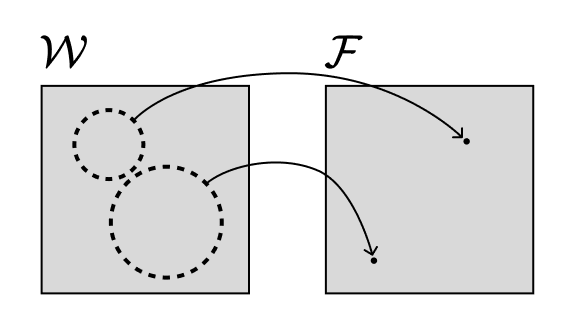When the parameter-function map is not one-to-one, the right object of study is not parameter space but function/distribution space.

Take the example of the Laplace approximation. If there's a local continuous symmetry in weight space, i.e., some direction you can walk that doesn't affect the probability density, then your density isn't locally Gaussian.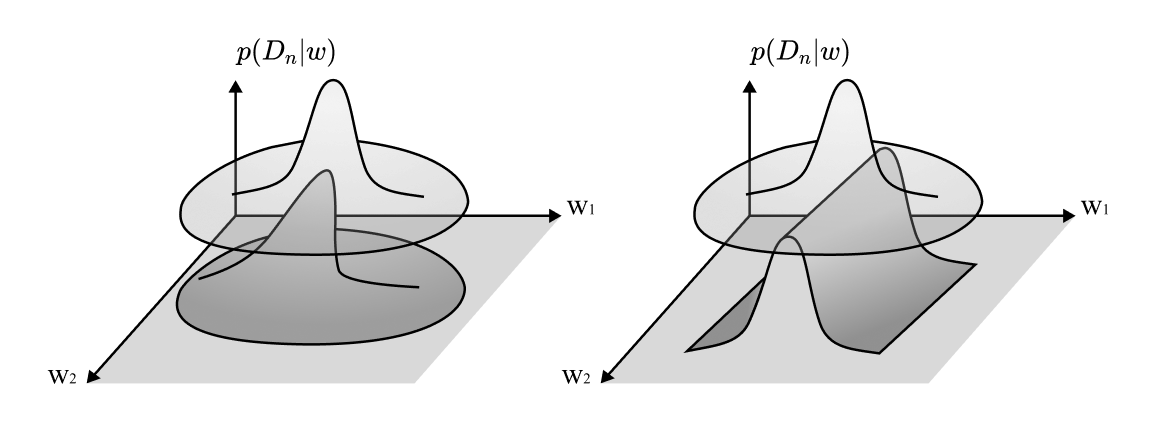The Laplace approximation breaks down when there is a direction of perfect flatness.

Even if the symmetries are non-continuous, the model will not in general be asymptotically normal. In other words, the standard central limit theorem does not hold.

The same problem arises if you're looking at loss landscapes in standard presentations of machine learning. Here, you'll find attempts to measure basin volume by fitting a paraboloid to the Hessian of the loss landscape at the final trained weights. It's the same trick, and it runs into the same problem.

This isn't the kind of thing you can just solve by adding a small  to the Hessian and calling it a day. There are ways to recover "volumes", but they require care. So, as a practical takeaway, if you ever find yourself adding  to make your Hessians invertible, recognize that those zero directions are important to understanding what's really going on in the network. Offer those eigenvalues the respect they deserve.

The consequence of these zeros (and, yes, they really exist in NNs) is that they reduce the effective dimensionality of your model. A step in these directions doesn't change the actual model being implemented, so you have fewer parameters available to "do things" with.

So the basic problem is this: almost all of the models we actually care about (not just neural networks, but Bayesian networks, HMMs, mixture models, Boltzmann machines, etc.) are loaded with symmetries, and this means we can't apply the conventional tooling of statistical learning theory.

# Learning is physics with likelihoods

Let's rewrite our beloved Bayes' update as follows,

where  is the negative log likelihood,

and ​ is the model evidence,

Notice that we've also snuck in an inverse "temperature", , so we're now in the tempered Bayes paradigm .

The immediate aim of this change is to emphasize the link with physics, where ​ is the preferred notation (and "partition function" the preferred name). The information theoretic analogue of the partition function is the free energy,

which will be the central object of our study.

Under the definition of a Hamiltonian (or "energy function"),

the connection is complete: statistical learning theory is just mathematical physics where the Hamiltonian is a random process given by the likelihood and prior. Just as the geometry of the energy landscape determines the behavior of the physical systems we study, the geometry of the log likelihood ends up determining the behavior of the learning systems we study.

In terms of this physical interpretation, the a posteriori distribution is the equilibrium state corresponding to this empirical Hamiltonian. The importance of the free energy is that it is the minimum of the free energy (not of the Hamiltonian) that determines the equilibrium.

Our next step will be to normalize these quantities of interest to make them easier to work with. For the negative log likelihood, this means subtracting its minimum value.

But that just gives us the KL divergence,

where ​ is the empirical entropy,

a term that is independent of .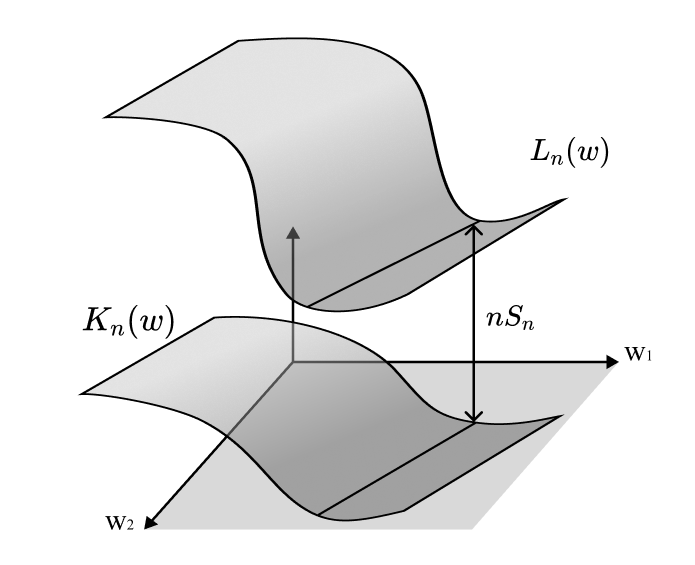The empirical Kullback-Leibler divergence is just a rescaled and shifted version of the negative log likelihood. Maximum likelihood estimation is equivalent to minimizing the empirical KL divergence.

Similarly, we normalize the partition function to get

and the free energy to get

This lets us rewrite the posterior as

The more important aim of this conversion is that now the minima of the term in the exponent, , are equal to 0. If we manage to find a way to express  as a polynomial, this lets us to pull in the powerful machinery of algebraic geometry, which studies the zeros of polynomials. We've turned our problem of probability theory and statistics into a problem of algebra and geometry.

# Why "singular"?

Singular learning theory is "singular" because the "singularities" (where the tangent is ill-defined) of the set of your loss function's minima,

determine the asymptotic form of the free energy. Mathematically, ​ is an algebraic variety, which is just a manifold with optional singularities where it does not have to be locally Euclidean.

By default, it's difficult to study these varieties close to their singularities. In order to do so anyway, we need to "resolve the singularities." We construct another well-behaved geometric object whose "shadow" is the original object in a way that this new system keeps all the essential features of the original.

It'll help to take a look at the following figure. The main idea behind resolution of singularities is to create a new manifold  and a map , such that  is a polynomial in the local coordinates of . We "disentangle" the singularities so that in our new coordinates they cross "normally".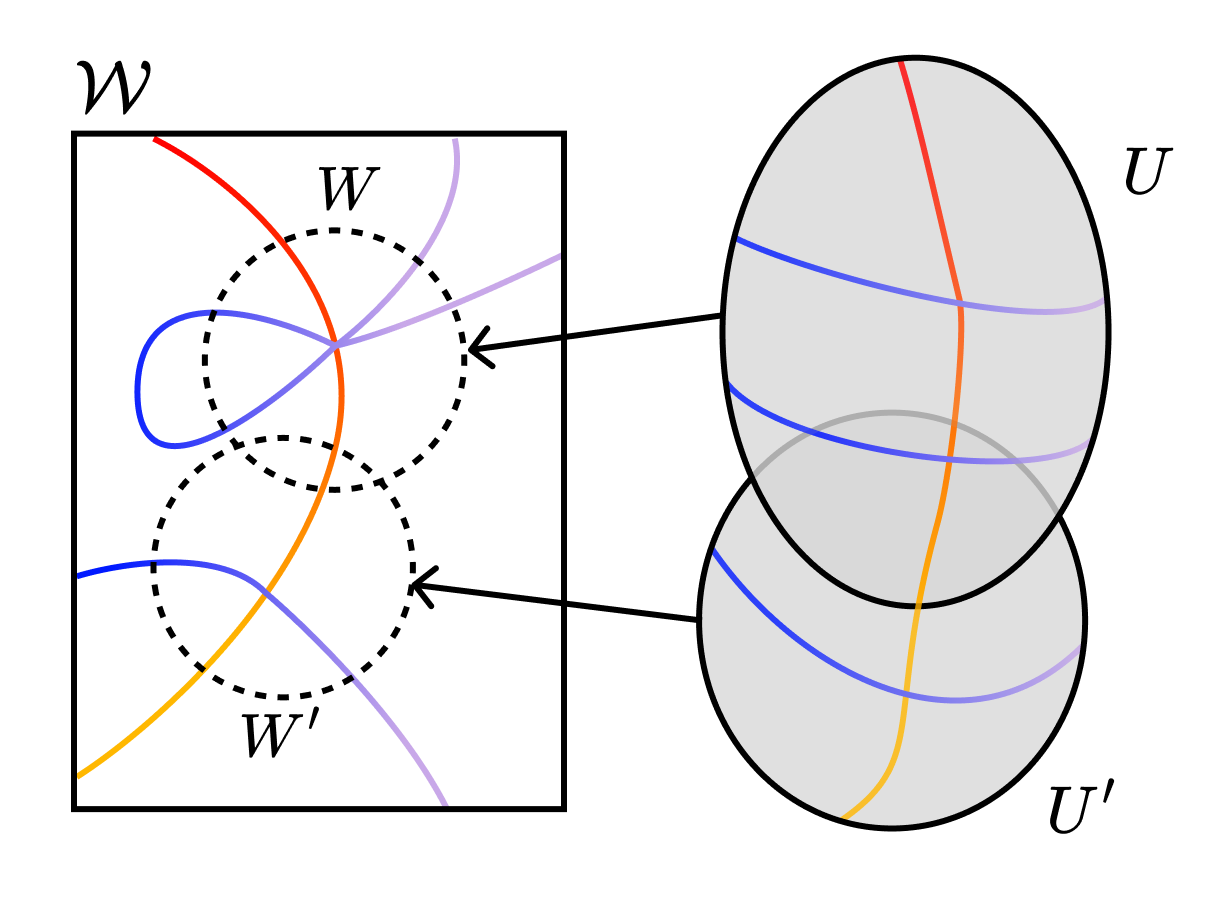Based on Figure 2.5 of . The lines represent the points that are in W0. The colors are just there to help you keep track of the points.

Because this "blow up" creates a new object, we have to be careful that the quantities we end up measuring don't change with the mapping — we want to find the birational invariants.

We are interested in one birational invariant in particular: the real log canonical threshold (RLCT). Roughly, this measures how "bad" a singularity is. More precisely, it measures the "effective dimensionality" near the singularity.

After fixing the central limit theorem to work in singular models, Watanabe goes on to derive the asymptotic form of the free energy as ,

where,   is the RLCT,  is the "multiplicity" associated to the RLCT,  is a (well-behaved) random variable, and  is a random variable that converges (in probability) to zero.

The important observation here is that the global behavior of your model is dominated by the local behavior of its "worst" singularities.

For regular (=non-singular) models, the RLCT is , and with the right choice of inverse temperature, the formula above simplifies to

which is just the BIC, as expected.

The free energy formula generalizes the BIC from classical learning theory to singular learning theory, which strictly includes regular learning theory as a special case. We see that singularities act as a kind of implicit regularization that penalizes models with higher effective dimensionality.

# Phase transitions are singularity manipulations

Minimizing the free energy is maximizing the model evidence, which, as we saw, is the preferred Bayesian way of doing model selection. Other paradigms may disagree, but at least among us this makes minimizing the free energy the central aim of statistical learning.

As in statistical learning, so in physics.

In physical systems, we distinguish microstates, such as the particular position and speed of every particle in a gas, with macrostates, such as the values of the volume and pressure. The fact that the mapping from microstates to macrostates is not one-to-one is the starting point for statistical physics: uniform distributions over microstates lead to much more interesting distributions over macrostates.

Often, we're interested in how continuously varying our levers (like temperature or the positions of the walls containing our gas) leads to discontinuous changes in the macroscopic parameters. We call these changes phase transitions.

The free energy is the central object of study because its derivatives generate the quantities we care about (like entropy, heat capacity, and pressure). So a phase transition means a discontinuity in one of the free energy's derivatives.

So too, in the setting of Bayesian inference, the free energy generates the quantities we care about, which are now quantities like the expected generalization loss,

Except for the fact that the number of samples, , is discrete, this is just a derivative.

So too, in learning, we're interested in how continuously changing either the model or the truth leads to discrete changes in the functions we implement and, thereby, to discontinuities in the free energy and its derivatives.

One way to subject this question to investigation is to study how our models change when we restrict our models to some subset of parameter space, . What happens when as vary this subset?

Recall that the free energy is defined as the negative log of the partition function. When we restrict ourselves to , we derive a restricted free energy,

which has a completely analogous asymptotic form (after swapping out the integrals over all of weight space with integrals over just this subset). The important difference is that the RLCT in this equation is the RLCT associated to the largest singularity in  rather than the largest singularity in .

What we see, then, is that phase transitions during learning correspond to discrete changes in the geometry of the "local" (=restricted) loss landscape. The expected behavior for models in these sets is determined by the largest nearby singularities.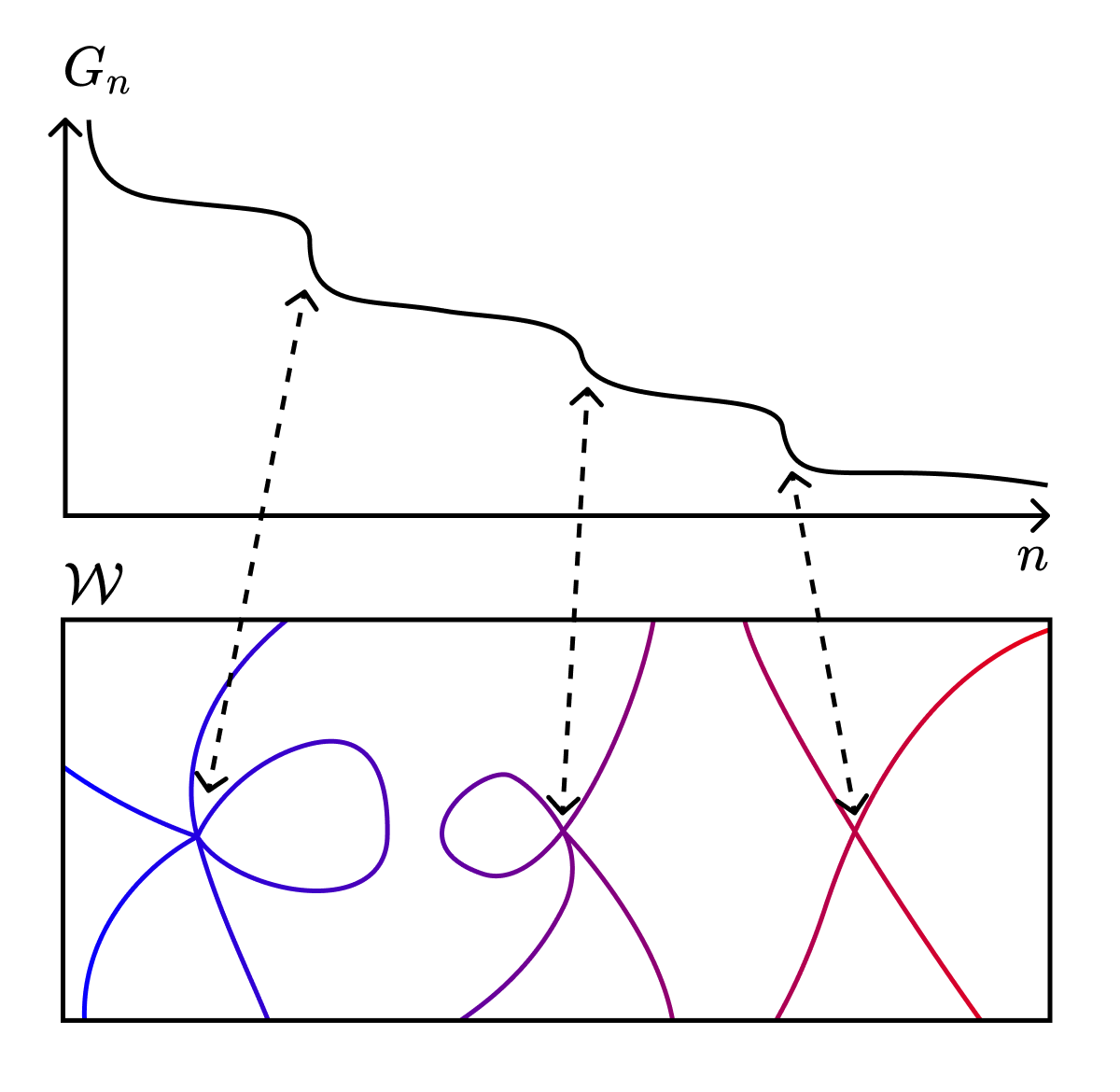In a Bayesian learning process, the relevant singularity becomes progressively simpler with more data. In general, learning processes involve trading off a more accurate fit against "regularizing" singularities. Based on Figure 7.6 in .

In this light, the link with physics is not just the typical arrogance of physicists asserting themselves on other people's disciplines. The link goes much deeper.

Physicists have known for decades that the macroscopic behavior of the systems we care about is the consequence of critical points in the energy landscape: global behavior is dominated by the local behavior of a small set of singularities. This is true everywhere from statistical physics and condensed matter theory to string theory. Singular learning theory tells us that learning machines are no different: the geometry of singularities is fundamental to the dynamics of learning and generalization.

# Neural networks are freaks of symmetries

The trick behind why neural networks generalize so well is something like their ability to exploit symmetry. Many models take advantage of the parameter-function map not being one-to-one. Neural networks take this to the next level.

There are discrete permutation symmetries, where you can flip two columns in one layer as long as you flip the two corresponding rows in the next layer, e.g.,

There are scaling symmetries associated to ReLU activations,

and associated to layer norm,

(Note: these are often broken by the presence of regularization.)

And there's a ​ symmetry associated to the residual stream (you can multiply the embedding matrix by any invertible matrix as long as you apply the inverse of that matrix before the attention blocks, the MLP layers, and the unembedding layer, and if you apply the matrix after each attention block and MLP layer).

But these symmetries aren't actually all that interesting. That's because they're generic. They're always present for any choice of . The more interesting symmetries are non-generic symmetries that depend on .

It's the changes in these symmetries that correspond to phase transitions in the posterior; this is the mechanism by which neural networks are able to change their effective dimensionality.

These non-generic symmetries include things like a degenerate node symmetry, which is the well-known case in which a weight is equal to zero and performs no work, and a weight annihilation symmetry in which multiple weights are non-zero but combine to have an effective weight of zero.

The consequence is that even if our optimizers are not performing explicit Bayesian inference, these non-generic symmetries allow the optimizers to perform a kind of internal model selection. There's a trade-off between lower effective dimensionality and higher accuracy that is subject to the same kinds of phase transitions as discussed in the previous section.

The dynamics may not be exactly the same, but it is still the singularities and geometric invariants of the loss landscape that determine the dynamics.

# Discussion and limitations

All of the preceding discussion holds in general for any model where the parameter-function mapping is not one-to-one. When this is the case, singular learning theory is less a series of interesting and debate-worthy conjectures than a necessary frame.

The more important question is whether this theory actually tells us anything useful in practice. Quantities like the RLCT are exceedingly difficult to calculate for realistic systems, so can we actually put this theory to use?

I'd say the answer is a tentative yes. Results so far suggest that the predictions of SLT hold up to experimental scrutiny — the predicted phase transitions are actually observable for small toy models.

That's not to say there aren't limitations. I'll list a few from here  and a few of my own.

Before we get to my real objections, here are a few objections I think aren't actually good objections:

• But we care about function-approximation. This whole discussion is couched in a very probabilistic context. In practice, we're working with loss functions and are approximating functions, not densities. I don't think this is much of a problem as it's usually possible to recover your Bayesian footing in deterministic function approximation. Even when this isn't the case, the general claim — that the geometry of singularities determine dynamics — seems pretty robust.
• But we don't even train to completion! (/We're not actually reaching the minimum loss solutions). I expect most of the results to hold for any level set of the loss landscape — we'll just be interested in the dominant singularities of the level sets we end up in (even if they don't perfectly minimize the loss).
• But calculating (and even approximating) the RLCT is pretty much intractable. In any case, knowing of something's theoretical existence can often help us out on what may initially seem like unrelated turf. A more optimistic counter would be something like "maybe we can compute this for simple one-layer neural networks, and then find a straightforward iterative scheme to extend it to deeper layers." And that really doesn't seem all too unreasonable — when I see all the stuff physicists can squeeze out of nature, I'm optimistic about what learning theorists can squeeze out of neural networks.
• But how do you adapt the results from  to realistic activations like swishes? In the same way that many of the universal approximation theorems don't depend on the particulars of your activation function, I don't expect this to be a major objection to the theory.
• But ReLU networks are not analytic. Idk man, seems unimportant.
• But what do asymptotic limits in  actually tell us about the finite case? I guess it's my background in statistical physics, but I'd say that a few trillion tokens is a heck of a lot closer to infinity than it is to zero. In all seriousness, physics has a long history of success with finite-size scaling and perturbative expansions around well-behaved limits, and I expect these to transfer.
• But isn't this all just a fancy way of saying it was broad basins this entire time? Yeah, so I owe you an apology for all the Hessian-shaming and introduction-clickbaiting. In practice, I do expect small eigenvalues to be a useful proxy to how well specific models can generalize — less than zeros, but not nothing. Overall, the question that SLT answers seems to be a different question: it's about why we should expect models on average (and up to higher order moments) to generalize.

My real objections are as follows:

• But these predictions of "generalization error" are actually a contrived kind of theoretical device that isn't what we mean by "generalization error" in the typical ML setting. Pretty valid, but I'm optimistic that we can find the quantities we actually care about from the ones we can calculate right now .
• But what does Bayesian inference actually have to do with SGD and its variants? This complaint seems rather important especially since I'm not sold on the whole NNs-are-doing-Bayesian-inference thing. I think it's conceivable that we can find a way to relate any process that decreases free energy to the predictions here, but this does remain my overall biggest source of doubt.
• But the true distribution is not realizable. For the above presentation, we assumed there is some choice of parameters ​ such that  is equal to  almost everywhere (this is "realizability" or "grain of truth"). In real-world systems, this is never the case. For renormalizable models, extending the results to the non-realizable case turns out to be not too difficult. For non-renormalizable theories, we're in novel territory.

# Where do we go from here?

I hope you've enjoyed this taster of singular learning theory and its insights: the sense of learning theory as physics with likelihoods, of learning as the thermodynamics of loss, of generalization as the presence of singularity, and of the deep, universal relation between global behavior and the local geometry of singularities.

The work is far from done, but the possible impact for our understanding of intelligence is profound.

To close, let me share one of directions I find most exciting — that of singular learning theory as a path towards predicting the scaling laws we see in deep learning models .

There's speculation that we might be able to transfer the machinery of the renormalization group, a set of techniques and ideas developed in physics to deal with critical phenomena and scaling, to understand phase transitions in learning machines, and ultimately to compute the scaling coefficients from first principles.

To borrow Dan Murfet's call to arms :

It is truly remarkable that resolution of singularities, one of the deepest results in algebraic geometry, together with the theory of critical phenomena and the renormalisation group, some of the deepest ideas in physics, are both implicated in the emerging mathematical theory of deep learning. This is perhaps a hint of the fundamental structure of intelligence, both artificial and natural. There is much to be done!

: Watanabe 2009

: Carroll 2021

: Metauni 2021-2023 (Super awesome online lecture series hosted in Roblox that you should all check out.)

: Guedj 2019

: Kaplan 2020

1. ^

The dimensionality of the optimal parameters also depends on the true distribution generating your distribution, but even if the set of optimal parameters is zero-dimensional, the presence of level sets elsewhere can still affect learning and generalization.

2. ^

And from the underlying true distribution.

3. ^

To be precise, this rests on the assumption of realizability — that there is some weight ​ for which  equals  almost everywhere. In this case, the minimum value of the negative log likelihood is the empirical entropy.

4. ^

They are, of course, wrong.

5. ^

So  is really a kind of inverse temperature, like . Increasing the number of samples decreases the effective temperature, which brings us closer to the (degenerate) ground state.

6. ^

A word with a specific technical sense but that is related to renormalization in statistical physics.

New Comment
1 comment, sorted by Click to highlight new comments since:

First of all, I really like the images, they made things easier to understand and are pretty. Good work with that!

My biggest problem with this is the unclear applicability of this to alignment. Why do we want to predict scaling laws? Doesn't that mostly promote AI capabilities, and not alignment very much?

Second, I feel like there's a confusion over several probability distributions and potential functions going on

• The singularities are those of the likelihood ratio
• We care about the generalization error with respect to some prior , but the latter doesn't have any effect on the dynamics of SGD or on what the singularity is
• The Watanabe limit ( as ) and the restricted free energy all are presented on results, which rely on the singularities, and somehow predict generalization. But all of these depend on the prior , and earlier we've defined the singularities to be of the likelihood function; plus SGD actually only uses the likelihood function for its dynamics.

What is going on here?

It's also unclear what the takeaway from this post is. How can we predict generalization or dynamics from these things? Are there any empirical results on this?

Some clarifying questions / possible mistakes:

is not a KL divergence, the terms of the sum should be multiplied by or .

the Hamiltonian is a random process given by the log likelihood ratio function

Also given by the prior, if we go by the equation just above that. Also where does "ratio" come from? Likelihood ratios we can find in the Metropolis-Hastings transition probabilities, but you didn't even mention that here. I'm confused.

But that just gives us the KL divergence.

I'm not sure where you get this. Is it from the fact that predicting p(x | w) = q(x) is optimal, because the actual probability of a data point is q(x) ? If not it'd be nice to specify.

the minima of the term in the exponent, K (w) , are equal to 0.

This is only true for the global minima, but for the dynamics of learning we also care about local minima (that may be higher than 0). Are we implicitly assuming that most local minima are also global? Is this true of actual NNs?

the asymptotic form of the free energy as

This is only true when the weights are close to the singularity right? Also what is , seems like it's the RLCT but this isn't stated